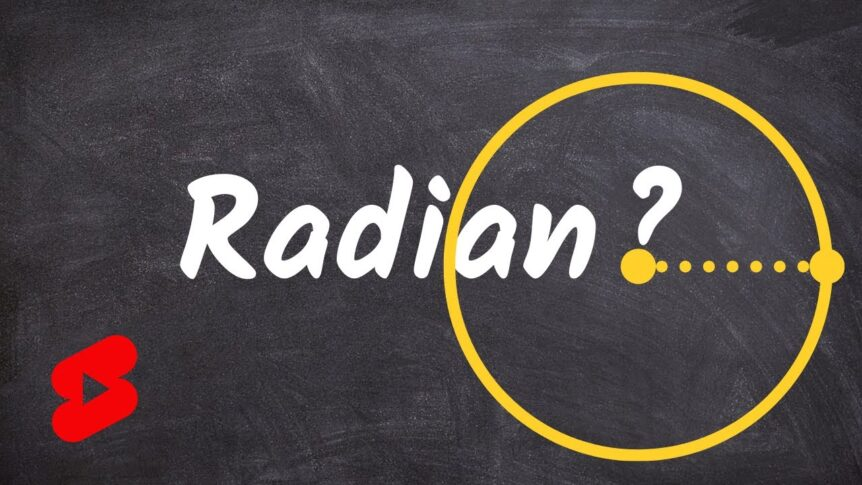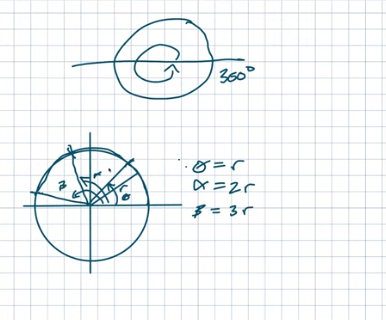# Where Does the Radian Come From?

The radian comes from a very similar origin starting from a circle. The radius is the distance from the center of a circle to any point on the circle. Theta, the measure of rotation is a radian. If I take this radius and wrap another one around so that I have two radians, it’s going to produce another measure of rotation. We can call it alpha, so alpha = two radians.θ = r         α = 2r         β = 3r

If I do this one more time, I get really close. I’m basically taking the radius and wrapping it around the circle three times. We’ll call this beta, and beta is equal to three radians. It almost looks like it gets halfway around the circle.